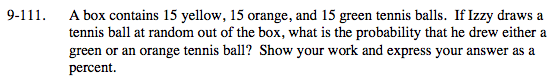### Home > MC2 > Chapter 9 > Lesson 9.3.3 > Problem9-111

9-111.$\text{Probability }= \frac{\text{number of tennis balls we're looking for}}{\text{Total number of tennis balls}}$

How many green and orange tennis balls are there?
How many total tennis balls are there?

15 green + 15 orange = 30 green or orange tennis balls.
15 green + 15 orange + 15 yellow = 45 total tennis balls.

$\frac{30}{45} \text{ Now convert this fraction to a percent.}$

$66 \frac{2}{3}$